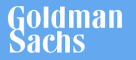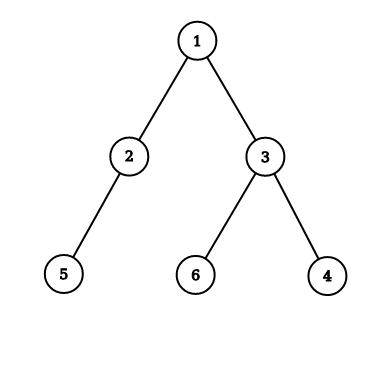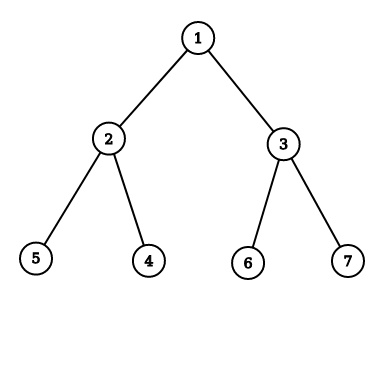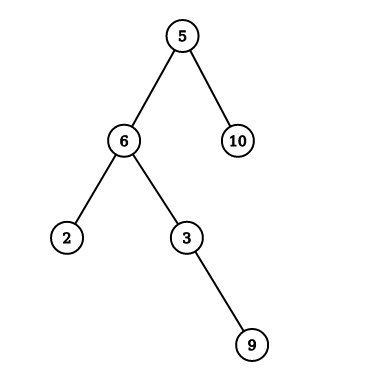New update is available. Click here to update.
Topics

# Level Order Traversal

Easy0/40
Average time to solve is 10m## Problem statement

You are given a ‘Binary Tree’.

Return the level-order traversal of the Binary Tree.

Example:
``````Input: Consider the following Binary Tree:
````````````Output:
Following is the level-order traversal of the given Binary Tree: [1, 2, 3, 5, 6, 4]
``````

Detailed explanation ( Input/output format, Notes, Images )
Sample Input 1:
``````1 2 3 5 4 6 7 -1 -1 -1 -1 -1 -1 -1 -1
``````

Sample Output 1:
``````1 2 3 5 4 6 7
``````

Explanation of Sample Input 1:
``````The Binary Tree given in the input is as follows:
``````Sample Input 2:
``````5 6 10 2 3 -1 -1 -1 -1 -1 9 -1 -1
``````

Sample Output 2:
``````5 6 10 2 3 9
``````

Explanation of Sample Input 2:
``````The Binary Tree given in the input is as follows:
``````Expected Time Complexity:
``````Try to do this in O(n).
``````

Constraints:
``````1 <= n <= 100000
where 'n' is the number of nodes in the binary tree.

Time Limit: 1 sec
``````Console